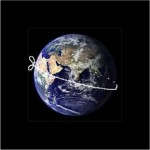A wire belt is tied tightly around the Earth at the equator. Suppose the belt is made exactly one metre longer and held around the Earth at the equator so that it is the same distance away from the Earth everywhere. Would a mouse be able to crawl under the new belt? How do you know?

Would the answer be the same for the moon?

Notes for Teachers – Belt Around The Earth.

See the related problems for younger learners:

SQUARE FENCE

NOT-SO-SQUARE FENCE

Tagged with:

### 2 Responses to Belt Round The World

1.toni says:

In the SA curriculum, finding the circumference of a circle is introduced for Grade 8 in term 3 (CAPS 4.1). This is a good problem for revision in grades 9 to 12 or as a challenging problem for high achievers in Grade 8.

The problem is essentially 2-dimensional.

Imagine cutting through the earth at the equator which is just a circle of radius R, where R is the radius of the earth. What is the circumference?

Then imagine the belt, also a circle, this time of radius R+d, where d is the distance between the belt and the ground. What is the circumference of this circle?

You know the belt is exactly 1 metre longer than the circumference of the earth.

Can you use these facts to solve this problem?

2.Joe Lehopa says:

looking at the fact that the distance around the earth is of the wire belt will be the same, I therefore am made to believe that the mouse will be able to crawl under the new belt cause it is enlarged.The same will apply to the moon.(spherical objects)